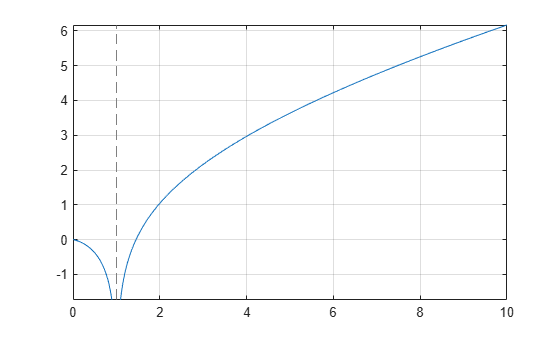Logarithmic integral function

## Syntax

``A = logint(x)``

## Description

example

````A = logint(x)` evaluates the logarithmic integral function (integral logarithm).```

## Examples

### Integral Logarithm for Numeric and Symbolic Arguments

`logint` returns floating-point or exact symbolic results depending on the arguments you use.

Compute integral logarithms for these numbers. Because these numbers are not symbolic objects, `logint` returns floating-point results.

`A = logint([-1, 0, 1/4, 1/2, 1, 2, 10])`
```A = 0.0737 + 3.4227i 0.0000 + 0.0000i -0.1187 + 0.0000i -0.3787 + 0.0000i... -Inf + 0.0000i 1.0452 + 0.0000i 6.1656 + 0.0000i```

Compute integral logarithms for the numbers converted to symbolic objects. For many symbolic (exact) numbers, `logint` returns unresolved symbolic calls.

`symA = logint(sym([-1, 0, 1/4, 1/2, 1, 2, 10]))`
```symA = [ logint(-1), 0, logint(1/4), logint(1/2), -Inf, logint(2), logint(10)]```

Use `vpa` to approximate symbolic results with floating-point numbers:

`A = vpa(symA)`
```A = [ 0.07366791204642548599010096523015... + 3.4227333787773627895923750617977i,... 0,... -0.11866205644712310530509570647204,... -0.37867104306108797672720718463656,... -Inf,... 1.0451637801174927848445888891946,... 6.1655995047872979375229817526695]```

### Plot Integral Logarithm

Plot the integral logarithm function on the interval from 0 to 10.

```syms x fplot(logint(x),[0 10]) grid on```### Handle Expressions Containing Integral Logarithm

Many functions, such as `diff` and `limit`, can handle expressions containing `logint`.

Find the first and second derivatives of the integral logarithm:

```syms x dA = diff(logint(x), x) dA = diff(logint(x), x, x)```
```dA = 1/log(x) dA = -1/(x*log(x)^2)```

Find the right and left limits of this expression involving `logint`:

`A_r = limit(exp(1/x)/logint(x + 1), x, 0, 'right')`
```A_r = Inf```
`A_l = limit(exp(1/x)/logint(x + 1), x, 0, 'left')`
```A_l = 0```

## Input Arguments

collapse all

Input, specified as a symbolic number, variable, expression, or function, or as a vector or matrix of symbolic numbers, variables, expressions, or functions.

collapse all

### Logarithmic Integral Function

The logarithmic integral function, also called the integral logarithm, is defined as follows:

`$\text{logint}\left(x\right)=\text{li}\left(x\right)=\underset{0}{\overset{x}{\int }}\frac{1}{\mathrm{ln}\left(t\right)}\text{\hspace{0.17em}}dt$`

## Tips

• `logint(sym(0))` returns `1`.

• `logint(sym(1))` returns `-Inf`.

• `logint(z) = ei(log(z))` for all complex `z`.

 Gautschi, W., and W. F. Cahill. “Exponential Integral and Related Functions.” Handbook of Mathematical Functions with Formulas, Graphs, and Mathematical Tables. (M. Abramowitz and I. A. Stegun, eds.). New York: Dover, 1972.

#### Mathematical Modeling with Symbolic Math Toolbox

Get examples and videos